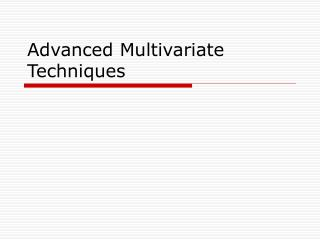DownloadDownload PresentationTélécharger la présentation- - - - - - - - - - - - - - - - - - - - - - - - - - - E N D - - - - - - - - - - - - - - - - - - - - - - - - - - -
##### Presentation Transcript

2. Factor Analysis • Purpose: define the underlying structure in a data matrix • Examines interrelationships among a large number of variables by defining a set of common underlying dimensions or factors • 1st identifies the separate dimensions of the structure • 2nd – determines the extent to which each variable is explained by each dimension • Utilized to summarize data and data reduction

3. Characteristics • Interdependence technique • All variables considered simultaneously • Utilizes at least interval-level data • Utilized either as exploratory or confirmatory • Exploratory – useful in searching the structure without any a priori constraints • Confirmatory – based upon preconceived hypotheses (theory) or prior data analysis

4. Using Factor Analysis • Principal Component Analysis • Considers total variance – with little unique or specific variance and/or error variance • Communalities – estimates of shared or common variance among the variables • # of Factors • Eigenvalues greater than 1.0 -- any individual factor should account for the variance of at least a single variable if it is to be retained for interpretation • A priori (confirmatory) • % of variance – ensure practical significance Social sciences – 60% of total variance is satisfactory

5. Using Factor Analysis • Interpretation • Unrotated factor matrix • Rotated factor matrix • Orthogonal – axes maintained at 90 degrees; 1st factor is viewed as the single best summary of the linear relationships while the next factors would be derived from the variance remaining after the 1st factor has been extracted – continues until all variance has been exhausted • Oblique – allow correlated factors instead of maintaining independence between the rotated factors

7. Interpretation of Factor Matrix • Examine rotated factor matrix • Identify the highest loading for each variable • Examine those variables that do NOT load on any one factor (delete items and/or expand factor solution) • Label factors • Validate the structure

8. Using Factor Analysis • Creating summated scales • Define – conceptual definition • Assess dimensionality • Calculate reliability • Assess validity

9. Discriminant Analysis • Categorical dependent variable (nominal or nonmetric) • Multiple metric (interval or ratio) independent variables • Involves deriving a variate – the linear combination of the two (or more) independent variables that will discriminate best between a priori defined groups • Achieved by setting the variate’s weights for each variable to maximize the between-group variance relative to the within-group variance • Tests the hypothesis that the group means for a set of independent variables for two or more groups are equal • A single composite discriminant z-score is obtained for each individual in the analysis and by averaging the z-scores, a group mean or centroid is determined • Statistical test is a generalized measure of the distance between the group centroids computed by comparing the distributions of the z-scores for the groups. If the overlap is small, the discriminant function separates the groups well; if the overlap is large, the function is a poor discriminator between the groups

10. Objectives of Discriminant Analysis • Determine if statistically significant differences exist between the average score profiles on a set of variables for two or more a priori defined groups • Determine which independent variable(s) account the most for differences between the groups • Establish procedures for classifying objects into groups based upon their scores • Establish the number and composition of the dimensions of discrimination between groups formed from the set of independent variables

11. Process • Select independent & dependent variables • Dependent variable – categorical; true categorical or created from ratio or interval-level data • Independent variables – metric • Use previous research, theory, and/or intuition

12. Process continued • Sensitive to sample size • Suggest 20 cases or observations per independent variable with a minimum of 5 observations per independent variable • Consider sample size of each group • Each group should have at least 20 observations • If groups vary in size, this can affect the discriminant function as well

13. Process continued • Division of the sample • Analysis sample • Hold-out sample

14. Estimating the Model • Simultaneous estimation – includes all IV at once and should be utilized when theory suggests all variables are important discriminators • Stepwise elimination – enters IV one at a time on the basis of their discriminating power

15. Assessing Fit • Calculate z-scores for each observation • Evaluating group differences • Mahalanobis D2 measure • Assess group membership prediction accuracy • Calculating a hit ratio – percentage correctly classified

16. Cluster Analysis • Purpose: Group objects based upon the characteristics they possess • Defines objects to maximize high internal (within cluster) homogeneity and high external (between cluster) heterogeneity • Cluster variate is the set of variables representing the characteristics used to compare objects

17. Method • Selection of variables • Determines inherent structure of the data as defined by the variables • Must consider theoretical, conceptual and practical implications • Method utilized • Deriving clusters & assessing model fit • Validating & profiling clusters